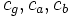# Direct factor implies central factor

This article gives the statement and possibly, proof, of an implication relation between two subgroup properties. That is, it states that every subgroup satisfying the first subgroup property (i.e., direct factor) must also satisfy the second subgroup property (i.e., central factor)
View all subgroup property implications | View all subgroup property non-implications

## Statement

### Verbal statement

Any direct factor of a group is a central factor.

### Statement with symbols

Suppose$H$ is a direct factor of a group$G$, i.e.,$H$ is a normal subgroup of$G$ and there exists a normal subgroup$K$ of$G$ such that$HK = G$ and$H \cap K$ is trivial. Then,$H$ is a central factor of$G$, i.e.,$HC_G(H) = G$.

## Facts used

1. Internal direct product implies internal central product

## Proof

### Proof using the product with centralizer definition

Given: A group$G$, normal subgroups$H,K$ of$G$ such that$HK = G$ and$H \cap K$ is trivial.

To prove:$HC_G(H) = G$.

Proof:

1. Every element of$H$ commutes with every element of$K$: For$h \in H$ and$k \in K$, the commutator$[h,k] = hkh^{-1}k^{-1}$ is in$H$ (because$H$ is normal) and is also in$K$ (because$K$ is normal). (This is based on one of the equivalent definitions of normal subgroup. It can also be seen by seeing that$[h,k] = h(kh^{-1}k^{-1}) = (hkh^{-1})k^{-1}$). Since$H \cap K$ is trivial, we obtain that$[h,k]$ is the identity element, so$hk = kh$.
2.$K \le C_G(H)$: This is a reformulation of the previous step.
3.$HC_G(H) = G$: Since$K \le C_G(H)$,$G = HK \le HC_G(H) \le G$. Equality holds throughout, so$HC_G(H) = G$.

Note that step (1) above is sometimes taken as part of the definition of internal direct product, in which case it does not need to be proved.

### Hands-off proof using direct product and central product

By fact (1), an internal direct product is an internal central product. A direct factor is a factor in an internal direct product, and a central factor is a factor in an internal central product. Thus, a direct factor must be a central factor.

### Proof using the inner automorphism definition of central factor

Given:A group$G$ that is an internal direct product of subgroups$H$ and$K$. In other words,$HK = G$ and$H \cap K$ is trivial.

To prove: For any$g \in G$, there exists$h \in H$ such that, restricted to$H$, conjugation by$g$ equals conjugation by$h$.

Proof: We can write$g = ab$ with$a \in H$,$b \in K$ since$G = HK$.

1. Every element of$H$ commutes with every element of$K$: For$h \in H$ and$k \in K$, the commutator$[h,k] = hkh^{-1}k^{-1}$ is in$H$ (because$H$ is normal) and is also in$K$ (because$K$ is normal). (This is based on one of the equivalent definitions of normal subgroup. It can also be seen by seeing that$[h,k] = h(kh^{-1}k^{-1}) = (hkh^{-1})k^{-1}$). Since$H \cap K$ is trivial, we obtain that$[h,k]$ is the identity element, so$hk = kh$.
2. Restricted to$H$, conjugation by$a$ equals conjugation by$g$: If$c_g, c_a, c_b$ denote conjugation by$g,a,b$ respectively, then ,math>c_g = c_a \circ c_b[/itex]. Restricting to$H$,$c_b$ is trivial by the previous step. Thus,$c_g = c_a$.